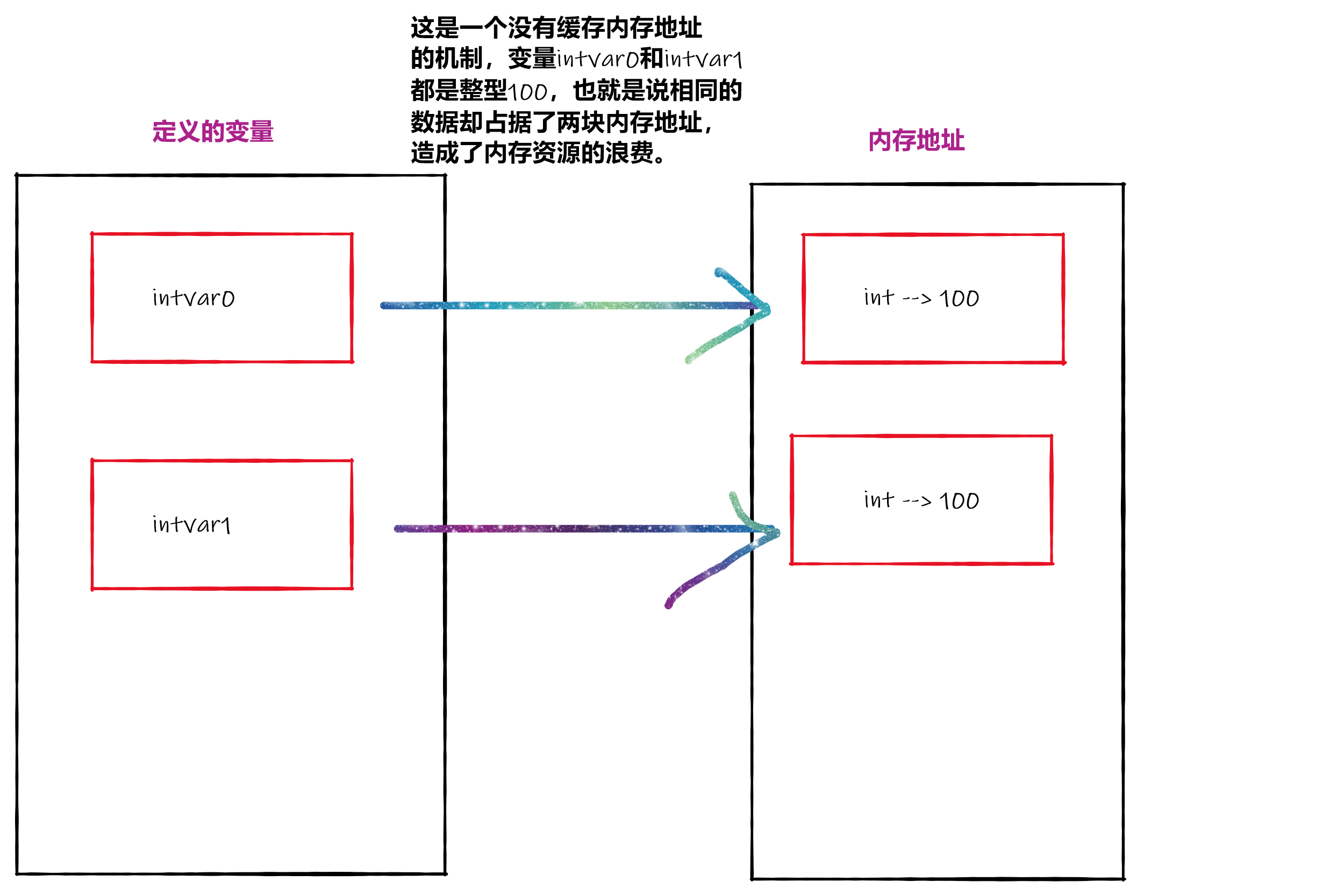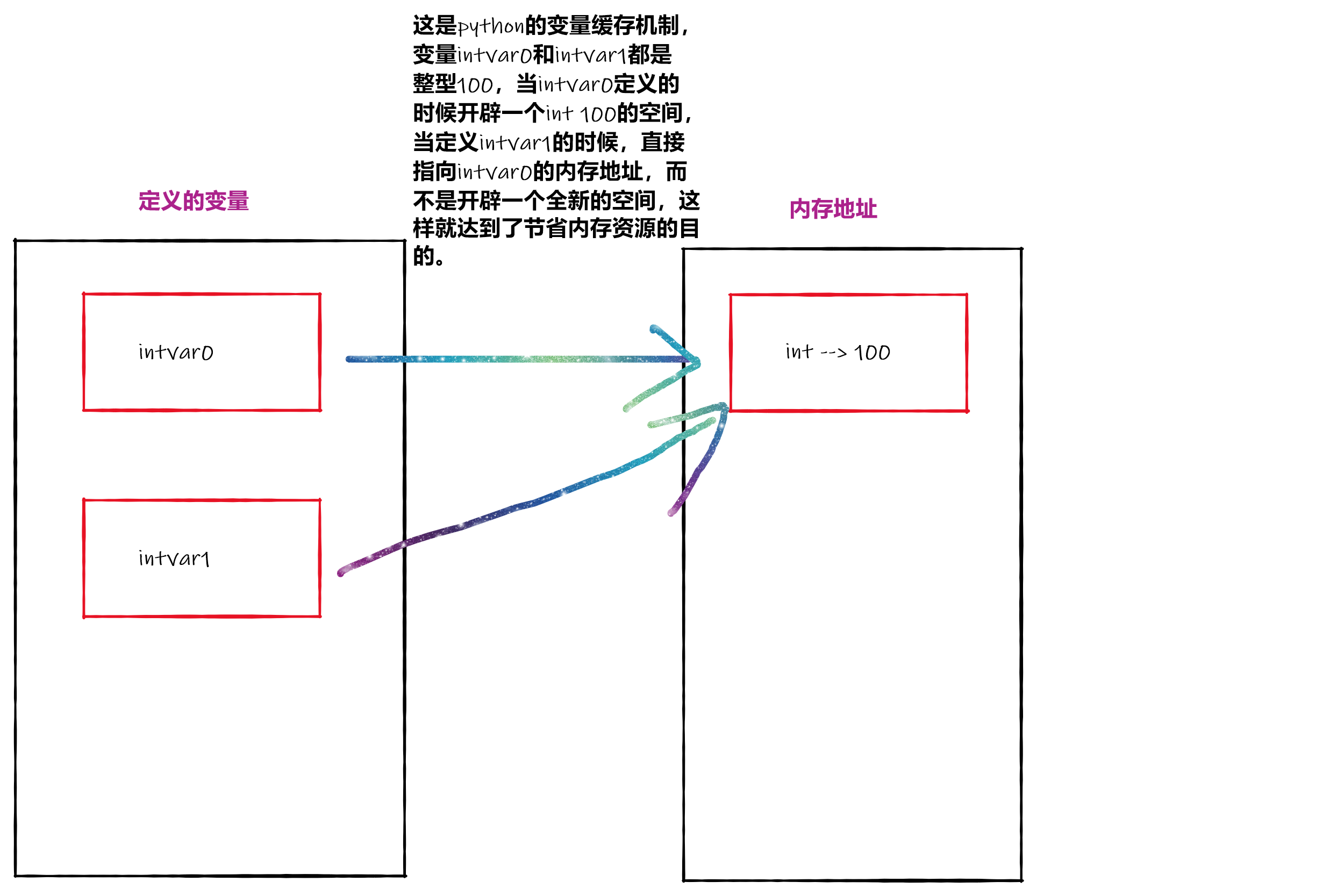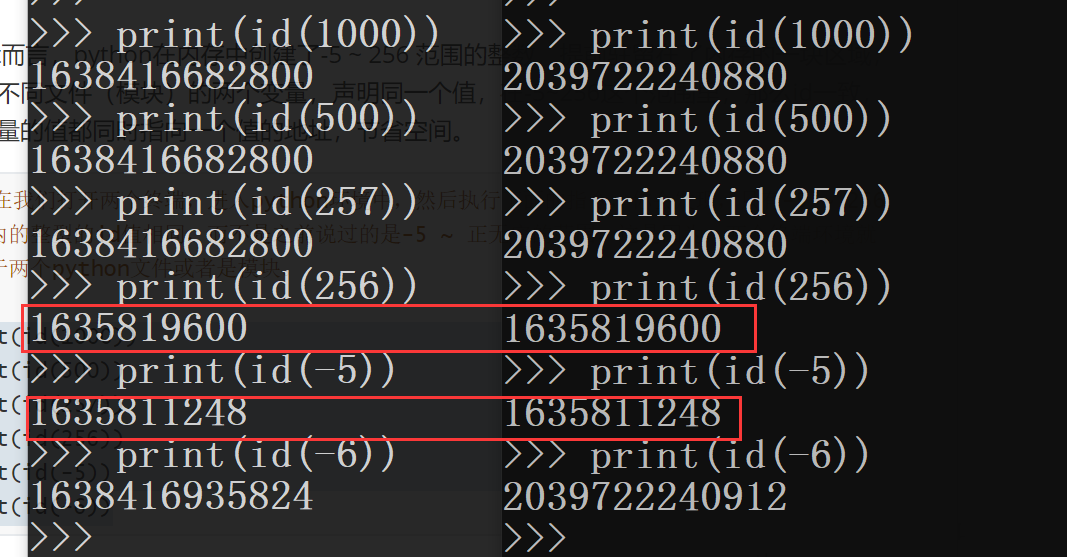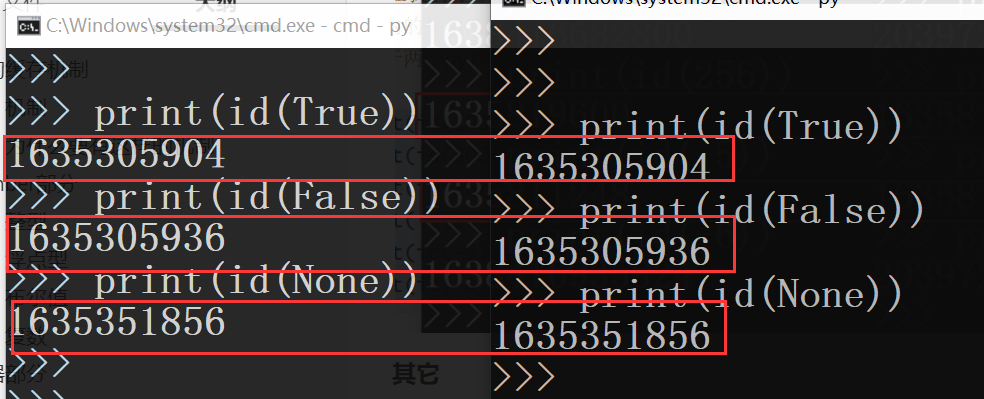python的变量缓存机制

变量的缓存机制

为什么要有这样的机制python中使用id()函数查看数据的内存地址

number部分

整型

# 在后续的版本中所有的数的id地址都一致

# 相同
print(id(9999999), id(9999999))
print(id(100), id(100))
print(id(-5), id(-5))

# 不同
print(id(-6), id(-6))
浮点型

# 相同
print(id(9999999.0), id(9999999.0))
print(id(100.0), id(100.0))

# 不同
print(id(-5.0), id(-5.0))
print(id(-6.0), id(-6.0))
布尔值

# 相同
print(id(True), id(True))
print(id(False), id(False))
复数

# 相同
print(id(1j), id(1j))
print(id(0j), id(0j))

# 不同
print(id(1234j), id(3456j))
print(id(1+1j), id(1+1j))
print(id(2+0j), id(2+0j))

容器部分

字符串

# 相同
print(id('hello '), id("hello "))

# 不同
print(id('msr'), id('wxd'))

1. 乘数为1：只要数据相同，地址就是相同的

# 等于1，和正常的情况下是一样的，只要值相同地址就是一样的
a = 'hello ' * 1
b = 'hello ' * 1
print(a is b)
a = '祖国' * 1
b = '祖国' * 1
print(a is b)

2. 乘数大于1：只有仅包含数字、字母、下划线时地址是相同的，而且字符串的长度不能大于20

# 纯数字字母下划线，且长度不大于20
a = '_70th' * 3
b = '_70th' * 3
c = '_70th_70th_70th'
print(a, id(a), len(a))
print(b, id(b), len(b))
print(c, id(c), len(c))
print(a is b is c)
'''
结果：
_70th_70th_70th 1734096389168 15
_70th_70th_70th 1734096389168 15
_70th_70th_70th 1734096389168 15
True
'''

# 纯数字字母下划线，长度大于20
a = 'motherland_70th' * 3
b = 'motherland_70th' * 3
c = 'motherland_70thmotherland_70thmotherland_70th'
print(a, id(a), len(a))
print(b, id(b), len(b))
print(c, id(c), len(c))
print(a is b is c)
'''
结果：
motherland_70thmotherland_70thmotherland_70th 2281801354864 45
motherland_70thmotherland_70thmotherland_70th 2281801354960 45
motherland_70thmotherland_70thmotherland_70th 2281801354768 45
False
'''

# 有其它字符，且长度不大于20
a = '你好' * 3
b = '你好' * 3
c = '你好你好你好'
print(a, id(a), len(a))
print(b, id(b), len(b))
print(c, id(c), len(c))
print(a is b is c)
'''
结果：
你好你好你好 3115902573360 6
你好你好你好 3115902573448 6
你好你好你好 3115900671904 6
False
'''

字符串指定驻留

from sys import intern

a = intern('祖国70华诞： my 70th birthday of the motherland' * 1000)
b = intern('祖国70华诞： my 70th birthday of the motherland' * 1000)
c = intern('祖国70华诞： my 70th birthday of the motherland' * 1000)
d = intern('祖国70华诞： my 70th birthday of the motherland' * 1000)
e = intern('祖国70华诞： my 70th birthday of the motherland' * 1000)

print(a is b is c is d is e)
元组

# 相同
print(id(()), id(tuple()))

# 不同
print(id((1, 2)), id((1, 2)))
列表、集合、字典

# 列表、非空元组、集合、字典 无论在声明情况下，id表示都不会相同

# 不同
print(id([]), id([]))
print(id(set()), id(set()))
print(id({}), id({}))

总结

# -->Number 部分
1.对于整型而言，-5~正无穷范围内的相同值 id一致
2.对于浮点数而言，非负数范围内的相同值 id一致
3.布尔值而言,值相同情况下，id一致
4.复数在 实数+虚数 这样的结构中永不相同(只有虚数的情况例外,只有虚数的虚数相同才会id一致)

# -->容器类型部分
5.字符串 和 空元组 相同的情况下，地址相同
6.列表，元组，字典，集合无论什么情况 id标识都不同 [空元组例外]

小数据池

小数据池支持什么类型

int

# 现在我们打开两个终端，进入python环境中，然后执行下面的指令，你会发现，只有-5 ~ 256范围内的整型的id值相同，而不是之前说过的是-5 ~ 正无穷的范围，这是因为，两个终端环境就相当于两个python文件或者是模块。

print(id(1000))
print(id(500))
print(id(257))
print(id(256))
print(id(-5))
print(id(-6))其它

# 开启两个终端测试吧
print(id(True))
print(id(False))
print(id(None))posted @ 2021-01-23 22:40  小小垂髫  阅读(74)  评论(0编辑  收藏  举报